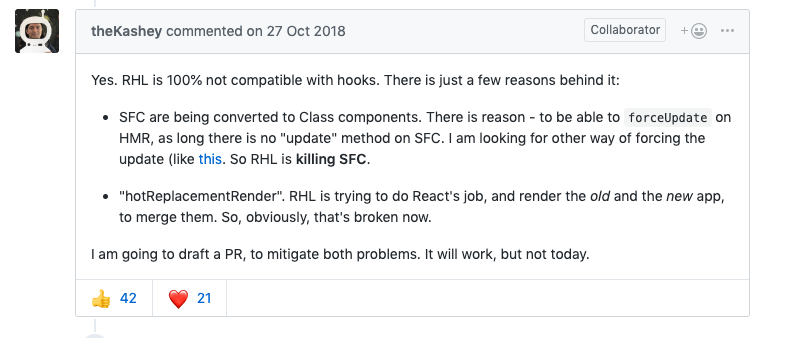# 【第三期】本周我们 37 人学了什么

## TS 中使用 `typeof` 关键字可以自动获取数据类型

``````javascript
const initialState = {
username: '',
mobile: '',
isVip: false,
addresses: [],
}

type IState = typeof initialState

class Comp extends Component<any istate> {
constructor(props) {
super(props);
this.state = {
...initialState
};
}
}
</any>``````

## 交换数组指定位置元素位置

``````js
// x , y是要交换元素的位置(index+1)
function arrIndexExchange(array, x, y){
array.splice(x - 1, 1, ...array.splice(y - 1, 1, array[x - 1]));
return array;
};
``````

## 位运算

• 用 "|" 取整
``````js
let num=1.5
num=num|0; // 1
``````
• 用 ">>" 取半
``````js
let num=4;
num=num>>1; // 2
``````
• 用 ">>" 加倍
``````js

let num=2;
num=num<<1; / / 4
``````
• 用 "^" 两值交换
``````js
let a=1;
let b=2;

a^=b;
b^=a;
a^=b;
// a===2,b===1
``````
• 用 "&" 判断奇数
``````js
let n=3;
let m=4;
n&1===1; // true 奇数
m&1===1; // false 偶数
``````
• 用 "~" 判断项是否存在
``````js
let firstname="Ma";
let fullname="Jack Ma";
let isExist=!!~fullname.indexOf(firstname); // true
``````

## arguments 的坑

• 当非严格模式中的函数 没有 包含 `剩余参数``默认参数``解构赋值`，那么arguments对象中的值会跟踪参数的值（反之亦然）
``````js

function sidEffecting(ary) {
ary = ary;
}
function bar(a,b,c) {
c = 10 // 注意这里，其实它修改的就是 arguments 对象里的参数
sidEffecting(arguments);
return a + b + c;
}
bar(1,1,1);   // 21
``````
• 当非严格模式中的函数 包含`剩余参数``默认参数``解构赋值`，那么arguments对象中的值不会跟踪参数的值（反之亦然）
``````js
function sidEffecting(ary) {
ary = ary;
}
function bar(a,b,c=3) {
c = 10
sidEffecting(arguments);
return a + b + c;
}
bar(1,1,1); // 12
``````

## 用canvas实现文字渐变效果

``````js
var context = canvas.getContext('2d')
var g = context.createLinearGradient(0,0,canvas.width,0)
g.addColorStop(0, 'red')
g.addColorStop(0.5, 'blue')
g.addColorStop(1, 'purple')
context.fillStyle = g
context.font = '36px fantasy'
context.fillText('hello canvas', 0, 100)
``````

## ES6 proxy 深度代理一个对象

``````js
function deepProxy(object, handler) {
if (isComplexObject(object)) {
addProxy(object, handler);
}
return new Proxy(object, handler);
}

function addProxy(obj, handler) {
for (let i in obj) {
if (typeof obj[i] === 'object') {
if (isComplexObject(obj[i])) {
addProxy(obj[i], handler);
}
obj[i] = new Proxy(obj[i], handler);
}
}
}

function isComplexObject(object) {
if (typeof object !== 'object') {
return false;
} else {
for (let prop in object) {
if (typeof object[prop] == 'object') {
return true;
}
}
}
return false;
}

let person = {
txt: 123,
name: 'tnt',
age: 26,
status: {
money: 'less',
fav: [1, 2, 3]
}
};
let proxyObj = deepProxy(person, {
get(target, key, receiver) {
console.log(`get--\${target}--\${key}`);
return Reflect.get(target, key);
},
set(target, key, value, receiver) {
console.log(`set--\${target}--\${key}-\${value}`);
return Reflect.set(target, key, value);
}
});
proxyObj.status.test = 13;
proxyObj.status.fav.push('33');
``````

## 格式化秒为 04:19 类型的小函数

``````js
const convertDuration = time => {
let minutes = Math.floor(time / 60);
let seconds = Math.floor(time - minutes * 60);
minutes = String(minutes).length < 2 ? String(minutes).padStart(2,'0'): minutes;
seconds = String(seconds).length < 2 ? String(seconds).padStart(2,'0'): seconds;
return minutes + ":" + seconds;
}

convertDuration(252); // "04:12"
``````

## hooks 与 react-hot-loader 冲突

``````javascript
Uncaught Error: Hooks can only be called inside the body of a function component.
``````

Hooks 确实有限制必须在函数组件中使用，但是我确实正确的使用了。于是搜索了一波资料，发现了一个 issus 出现了与我一样的问题：Incompatibility with react-hot-loader## 最后

• 点赞
• 写回答
• 关注问题
• 收藏
• 复制链接分享
• 邀请回答

#### 4条回答

• 格式化秒为 04:19 类型的小函数有个小问题，如果时间正好为6000秒时，时间则为00：00了。

点赞 评论 复制链接分享
• ``````js
const convertDuration = time => {
let minutes = Math.floor(time / 60);
let seconds = Math.floor(time - minutes * 60);
minutes = String(minutes).length < 2 ? String(minutes).padStart(2,'0'): minutes;
seconds = String(seconds).length < 2 ? String(seconds).padStart(2,'0'): seconds;
return minutes + ":" + seconds;
};

convertDuration(308); // 05:08
convertDuration(6000); // 100:00
``````
点赞 评论 复制链接分享
• ``````js
const convertDuration = time => {
let minutes = Math.floor(time / 60);
let seconds = Math.floor(time - minutes * 60);
minutes = String(minutes).length < 2 ? String(minutes).padStart(2,'0'): minutes;
seconds = String(seconds).length < 2 ? String(seconds).padStart(2,'0'): seconds;
return minutes + ":" + seconds;
};
<p>convertDuration(308); // 05:08
convertDuration(6000); // 100:00
</p>``````

已更新，感谢！

点赞 评论 复制链接分享
• `````` js
// x , y是要交换元素的位置(index+1)
function arrIndexExchange(array, x, y){
array.splice(x - 1, 1, ...array.splice(y - 1, 1, array[x - 1]));
return array;
};
``````
复杂度爆炸
点赞 评论 复制链接分享